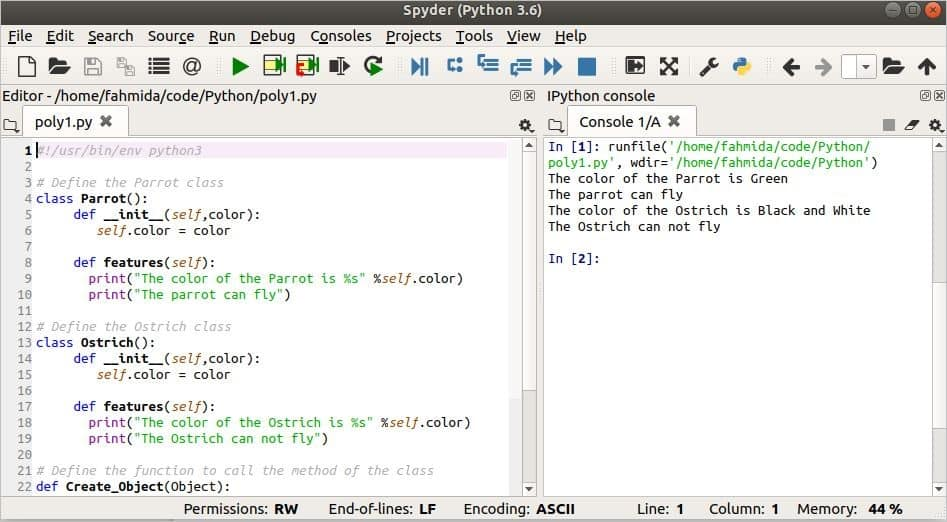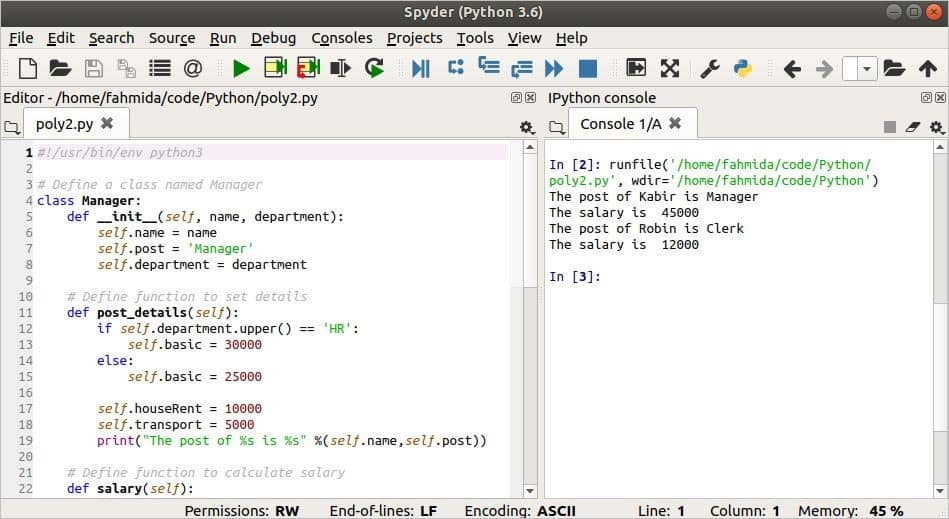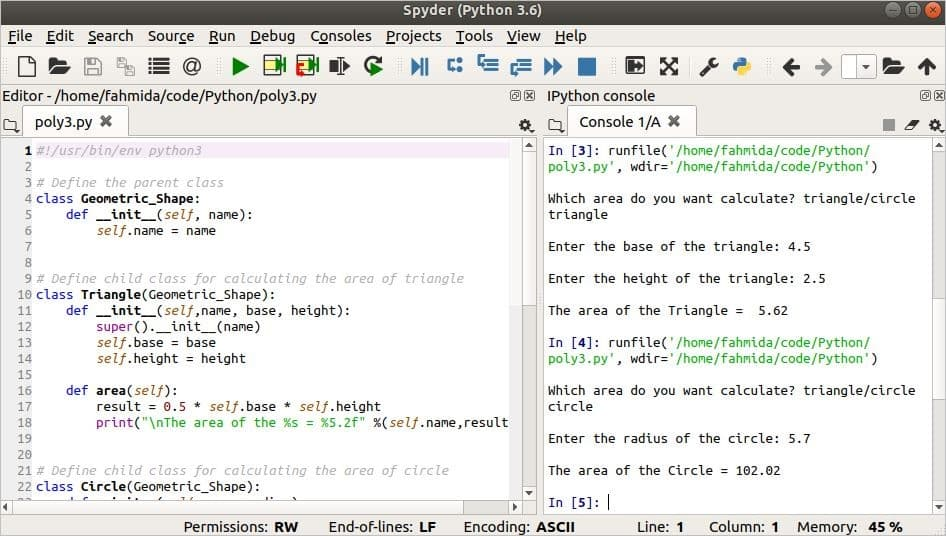Python

# Polymorphism in Python

Polymorphism means “many forms.” Polymorphism an important feature of Object-Oriented Programming (OOP).  When the same method is declared multiple times, for multiple purposes, and in different classes, then it is called polymorphism. Another feature of OOP is inheritance, through which the child class can be created by inheriting the features of the parent class. Sometimes, it requires the programmer to declare a method of the same name in both the parent and child classes for various purposes. This type of task can also be implemented by using polymorphism. This article explains how polymorphism can be defined in object-oriented programming (OOP).

### Example 1: Polymorphism with Functions and Objects

The following script shows the use of polymorphism between two different classes. A function is used to create the object of those classes. The value of the variable named color is initialized in the __init__() method of both the ‘Parrot’ and the ‘Ostrich’ classes at the time of object creation. The features() method is defined in both classes, but the output of the method for each class is a little bit different. The Create_Object() function is used to create an object of the class. This function is executed twice to create the object in the ‘Parrot’ class and  in the ‘Ostrich’ class. Each will call the features() method of both classes and print the output.

#!/usr/bin/env python3

# Define the Parrot class
class Parrot():
def __init__(self,color):
self.color = color

def features(self):
print("The color of the Parrot is %s" %self.color)
print("The parrot can fly")

# Define the Ostrich class
class Ostrich():
def __init__(self,color):
self.color = color

def features(self):
print("The color of the Ostrich is %s" %self.color)
print("The Ostrich can not fly")

# Define the function to call the method of the class
def Create_Object(Object):
Object.features()

# Create object of Parrot class
Create_Object(Parrot('Green'))
# Create object of Ostrich class
Create_Object(Ostrich('Black and White'))

Output

The following output shows that the object of the ‘Parrot’ class is created with ‘Green‘ as the color value. The function prints the output by calling the features() method of the ‘Parrot’ class. Next, the object of the ‘Ostrich’ class is created with ‘Black and White‘ as the color value. The function prints the output by calling the features() method of the ‘Ostrich’ class.### Example 2: Polymorphism in Unrelated Class Methods

Like in the previous example, the following script shows the use of polymorphism in two different classes, but no custom function is used to declare the object. The __init__() method of both the ‘Manager’ and ‘Clerk’ classes will initialize the necessary variables. Polymorphism is implemented here by creating the post_details() and salary() methods inside both classes. The content of these methods is different for each of these classes. Next, the object variables are created for both classes and iterated by a for a loop. In each iteration, the post_details() and salary() methods are called to print the output.

#!/usr/bin/env python3

# Define a class named Manager
class Manager:
def __init__(self, name, department):
self.name = name
self.post = 'Manager'
self.department = department

# Define function to set details
def post_details(self):
if self.department.upper() == 'HR':
self.basic = 30000
else:
self.basic = 25000

self.houseRent = 10000
self.transport = 5000
print("The post of %s is %s" %(self.name,self.post))

# Define function to calculate salary
def salary(self):
salary = self.basic + self.houseRent + self.transport
return salary

# Define a class named Clerk
class Clerk:
def __init__(self, name):
self.name = name
self.post = 'Clerk'

# Define function to set details
def post_details(self):
self.basic = 10000
self.transport = 2000
print("The post of %s is %s" %(self.name,self.post))

# Define function to calculate salary
def salary(self):
salary = self.basic + self.transport
return salary

# Create objects for the classes
manager = Manager("Kabir", "hr")
clerk = Clerk("Robin")

# Call the same functions from the different classes
for obj in (manager, clerk):
obj.post_details()
print("The salary is ",obj.salary())

Output

The following output shows that the object of the ‘Manger’ class is used in the first iteration of the for loop and the salary of the manager is printed after calculation. The object of the ‘Clerk’ class is used in the second iteration of the for loop and the salary of the clerk is printed after calculation.### Example 3: Polymorphism in Related Class Methods

The following script shows the use of polymorphism between two child classes. Here, both ‘Triangle’ and ‘Circle’ are the child classes of the parent class named ‘Geometric_Shape.’ According to the inheritance, the child class can access all the variables and methods of the parent class. The __init__() method of the ‘Geometric_Shape’ class is used in both child classes to initialize the variable name by using the super() method. The values of the base and height of the ‘Triangle’ class will be initialized at the time of object creation. In the same way, the radius values of the ‘Circle’ class will be initialized at the time of object creation. The formula for calculating the area of a triangle is ½ × base × height, which is implemented in the area() method of  the ‘Triangle’ class. The formula for calculating the area of a circle is 3.14 × (radius)2, which is implemented in the area() method of the ‘Circle’ class. The names of both methods are the same, here, but the purpose is different. Next, a string value will be taken from the user to create an object and to call the method based on the value. If the user types ‘triangle,’ then an object of the ‘Triangle’ class will be created, and if the user types ‘circle,’ then an object of the ‘Circle’ class will be created. If the user types any text without ‘triangle’ or ‘circle,’ then no object will be created, and an error message will be printed.

#!/usr/bin/env python3

# Define the parent class
class Geometric_Shape:
def __init__(self, name):
self.name = name

# Define child class for calculating the area of triangle
class Triangle(Geometric_Shape):
def __init__(self,name, base, height):
super().__init__(name)
self.base = base
self.height = height

def area(self):
result = 0.5 * self.base * self.height
print("\nThe area of the %s = %5.2f" %(self.name,result))

# Define child class for calculating the area of circle
class Circle(Geometric_Shape):
super().__init__(name)

def area(self):
print("\nThe area of the %s = %5.2f" %(self.name,result))

cal_area=input("Which area do you want calculate? triangle/circle\n")

if cal_area.upper()== 'TRIANGLE':
base = float(input('Enter the base of the triangle: '))
height = float(input('Enter the height of the triangle: '))
obj = Triangle('Triangle',base,height)
obj.area()
elif cal_area.upper()== 'CIRCLE':
obj.area()
else:
print("Wrong input")

Output

In the following output, the script is executed twice. The first time, triangle is taken as the input and the object is initialized by three values, ‘Triangle’, base, and height. These values are then used to calculate the area of the triangle and the output will be printed. The second time, circle is taken as input, and the object is initialized by two values, ‘Circle’ and radius. These values are then used to calculate the area of the circle and the output will be printed.### Conclusion

This article used easy examples to explain three different uses of polymorphism in Python. The concept of polymorphism can also be applied without classes, a method which is not explained here. This article helped readers to learn more about how to apply polymorphism in object-oriented based Python programming.